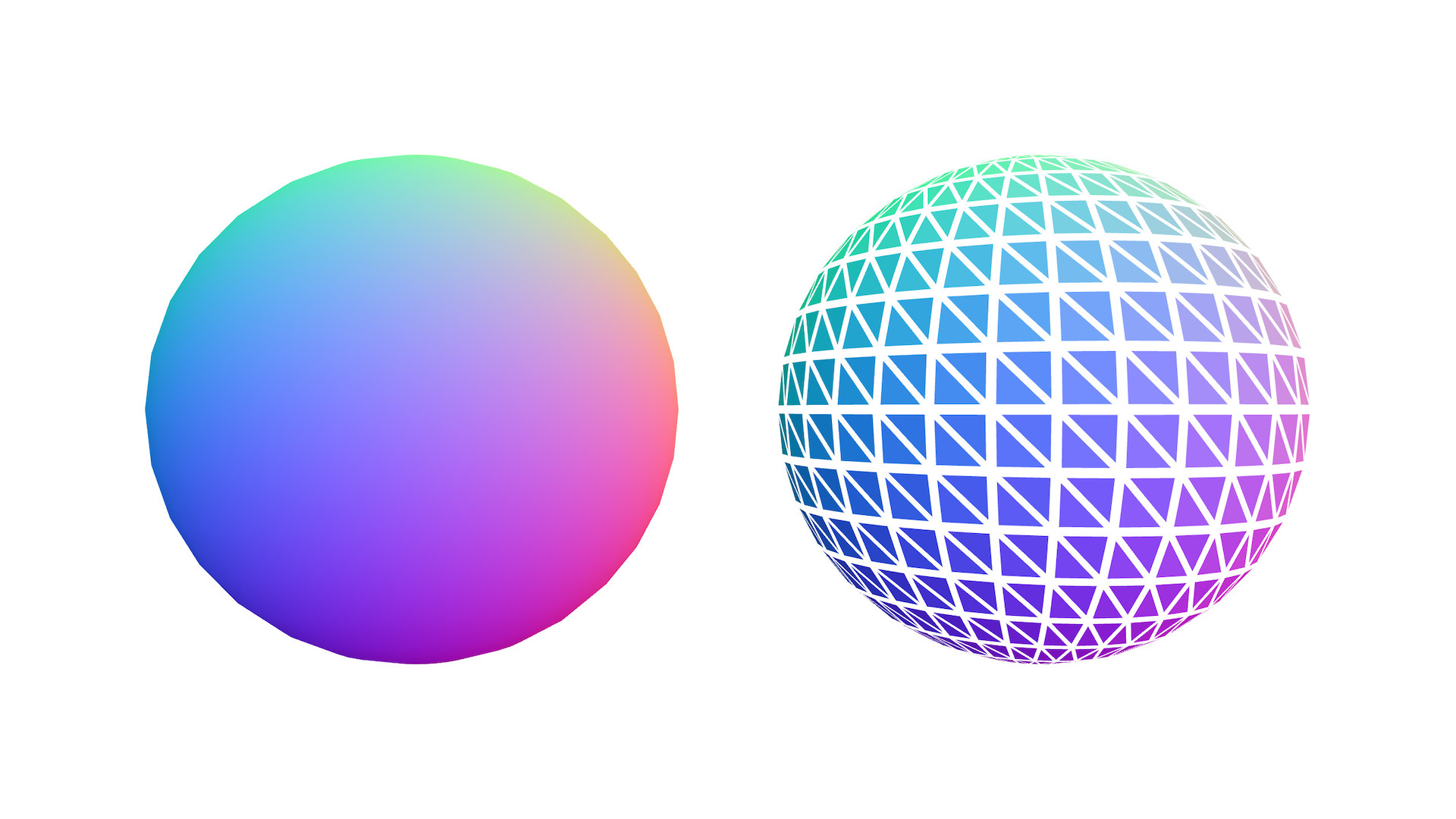# geom-split-vertices

Creates new geometry with unique vertices for each face.

Useful for flat shading.

## Usage

``````var splitVertices = require('geom-split-vertices')
var sphere = require('primitive-sphere')()

var g = splitVertices(sphere)
// g = { positions: [], cells: [] }
``````

## API

### `splitVertices(geometry)`

• `geometry` - geometry object { positions: [], cells: [] }

Returns new geometry with unique vertex position for each cell / face.

Note: Other vertex attributes like normals and uvs are lost and need to be recomputed.